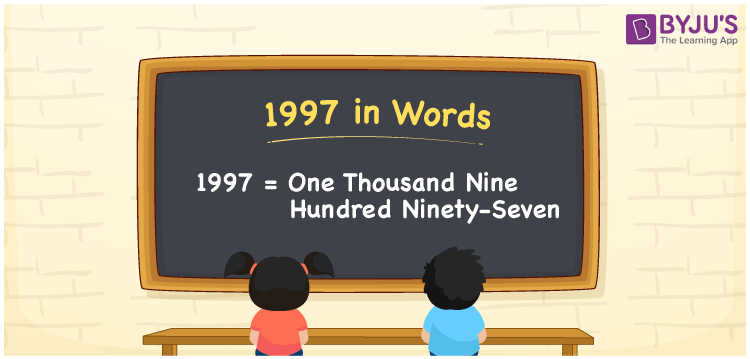# 1997 in Words

1997 in words is written as One thousand nine hundred ninety-seven. In both the International System of Numerals and the Indian System of Numerals, 1997 is written as One thousand nine hundred ninety-seven. The number 1997 is a Cardinal Number as it represents some quantity. For example, “the train tickets cost 1997 rupees”.

 1997 in Words One thousand nine hundred ninety-seven One thousand nine hundred ninety-seven in Number 1997

## 1997 in English Words

1997 in English words is read as “One thousand nine hundred ninety-seven.”## How to Write 1997 in Words?

To write 1997 in words, we shall use the place value chart. In the place value chart, put 1 in the thousands, 9 in the hundreds and tens, and 7 in the ones, respectively. Let us make a place value chart to write the number 1997 in words.

 Thousands Hundreds Tens Ones 1 9 9 7

Thus, we can write the expanded form as

1 × Thousand + 9 × Hundred + 9 × Ten + 7 × One

= 1 × 1000 + 9 × 100 + 9 × 10 + 7 × 1

= 1000 + 900 + 90 + 7

= 1997

= One thousand nine hundred ninety-seven.

1997 is a natural number between 1996 and 1998.

1997 in words – One thousand nine hundred ninety-seven

• Is 1997 an odd number? – Yes
• Is 1997 an even number? – No
• Is 1997 a perfect square number? – No
• Is 1997 a perfect cube number? – No
• Is 1997 a prime number? – Yes
• Is 1997 a composite number? – No

## Frequently Asked Questions on 1997 in Words

Q1

### How to write 1997 in words?

1997 in words is written as One thousand nine hundred ninety-seven.
Q2

### How to write 1997 in words in the International and Indian System of Numerals?

In both, the system of numerals, 1997 in words, is written as One thousand nine hundred ninety-seven.
Q3

### How to write 1997 in a place value chart?

In the place value chart, write 1 in the thousands, 9 in the hundreds and tens, and 7 in the ones, respectively.